# KSEEB Solutions for Class 7 Maths Chapter 2 Fractions and Decimals Ex 2.1

Students can Download Class 7 Maths Chapter 2 Fractions and Decimals Ex 2.1 Questions and Answers, Notes Pdf, KSEEB Solutions for Class 7 Maths helps you to revise the complete Karnataka State Board Syllabus and to clear all their doubts, score well in final exams.

## Karnataka State Syllabus Class 7 Maths Chapter 2 Fractions and Decimals Ex 2.1

Learn how to calculate if 596/652 and 365/838 are proportional ratios.

Question 1.
Solve:
I) 2 – $$\frac{3}{5}$$
Solution: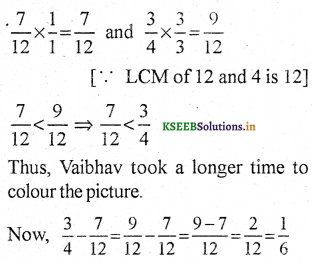ii) 4 + $$\frac{7}{8}$$
Solution: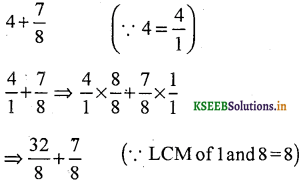iii) $$\frac{3}{5}+\frac{2}{7}$$
Solution: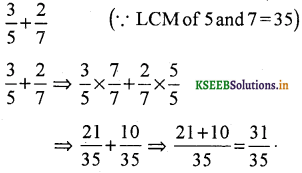iv) $$\frac{9}{11}-\frac{4}{15}$$
Solution: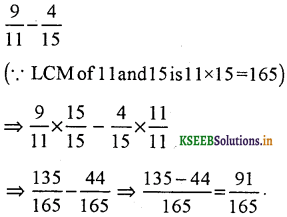v)Solution: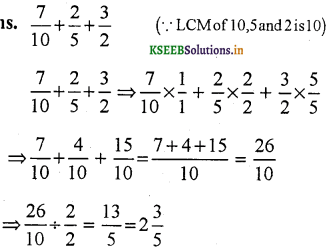vi)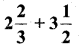Solution:
⇒ convert mixed fractions to improper fraction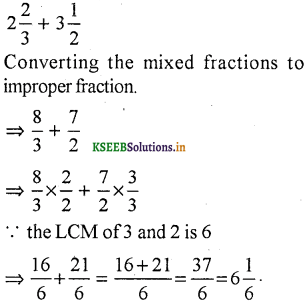Multiplying fractions with whole numbers calculator is a free online tool that gives the product of fractional and whole numbers for the given input.

vii)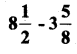Solution:
convert mixed fractions to improper fractionQuestion 2.
Arrange the following in descending order:
I)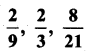Solution:
= We need to arrange these in descending order, To find which number is greater or smaller, we make their denominators equal.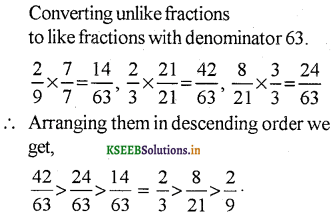ii)Solution:
⇒ We make their denominators equal, to find the descending order.Question 3.
In a “magic square”, the sum of the numbers in each row, in each column and along the diagonals is the same. Is this a magic square ?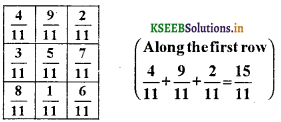Solution:
For Row,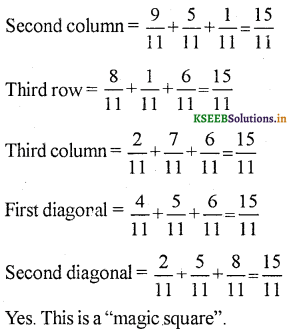Question 4.
A rectangular sheet of paper is 12$$\frac{1}{2}$$ cm long and 10$$\frac{2}{3}$$ cm wide. Find its perimeter.
Solution:
Length of rectangular sheet of paper = 12$$\frac{1}{2}$$ cm
(breadth) width of rectangular sheet paper = 10$$\frac{2}{3}$$ cm
Perimeter of rectangle = 2 (length + breadth)∴ Perimeter of the rectangular sheet of paper is $$46 \frac{1}{3}$$ cm.

Question 5.
Find the perimeters of
(a) ∆ ABE
(b) the rectangle BCDE in this figure. Whose perimeter is greater?Solution: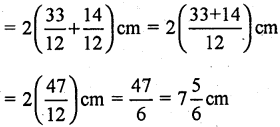Question 6.
Salil wants to put a picture in a frame. The picture is 7$$\frac{3}{10}$$ cm wide. How much should the picture be trimmed?
Solution:
We have,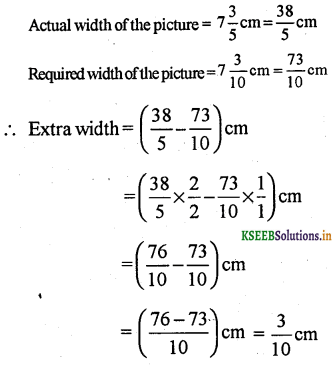Hence, $$\frac{3}{10}$$ cm width of the picture should be trimmed.Question 7.
Ritu ate $$\frac{3}{5}$$ part of an apple and the remaining apple was eaten by her brother Somu. How much part of the apple did Somu eat ? Who had the larger share? By how much?
Solution:
Total part = 1
Part eaten by Ritu = $$\frac{3}{5}$$
Part of the apple eaten by Somu = $$1-\frac{3}{5}$$
= $$\frac{5}{5}-\frac{3}{5}=\frac{5-3}{5}=\frac{2}{5}$$
Since $$\frac{3}{5}>\frac{2}{5}$$ , Ritu had the larger share.
Now, $$\frac{3}{5}-\frac{2}{5}=\frac{3-2}{5}=\frac{1}{5}$$
∴ Ritu had( $$\frac{1}{5}$$ ) part more then Somu.

Question 8.
Michael finished colouring in $$\frac{7}{12}$$ hour. Vaibhav finished colouring the same picture in $$\frac{3}{4}$$ hour. Who worked longer? By what fraction was it longer?
Solution:
Michael finished work in = $$\frac{7}{12}$$ hour
Vaibhav finished work in = $$\frac{3}{4}$$
We need to find who worked longer.
i.e., we need to find greater of = $$\frac{7}{12}$$ & $$\frac{3}{4}$$
We make their denominators equalHence, Vaibhav took $$\frac{1}{6}$$ hour more then minchel.